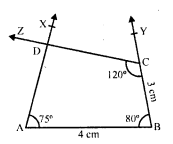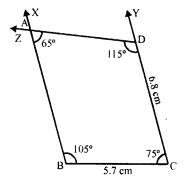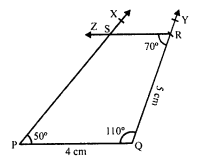## RD Sharma Class 8 Solutions Chapter 18 Practical Geometry Ex 18.5

These Solutions are part of RD Sharma Class 8 Solutions. Here we have given RD Sharma Class 8 Solutions Chapter 18 Practical Geometry Ex 18.5

Other Exercises

Question 1.
Construct a quadrilateral ABCD given that AB = 4 cm, BC = 3 cm, ∠A = 75°, ∠B = 80° and ∠C = 120°.
Solution:
Steps of construction :
(i) Draw a line segment AB = 4 cm.(ii) At A draw a ray AX making an angle of 75°.
(iii) At B draw another ray BY making an angle of 80° and cut off BC = 3 cm.
(iv) At C, draw another ray CZ making an angle of 120° which intersects AX at D.
Then ABCD is the required quadrilateral.

Question 2.
Construct a quadrilateral ABCD where AB = 5.5 cm, BC = 3.7 cm, ∠A = 60°, ∠B = 105° and ∠D = 90°.
Solution:
∠A = 60°, ∠B = 105° and ∠D = 90°
But ∠A + ∠B + ∠C + ∠D = 360° (Sum of angles of a quadrilateral)
⇒ 60° + 105° + ∠C + 90° = 360°
⇒ 255° + ∠C = 360°
⇒ ∠C = 360° – 255° = 105°
Steps of construction :
(i) Draw a line segment AB = 5.5 cm.
(ii) At A, draw a ray AX making an angle of(iii) At B, draw another ray BY making an angle of 105° and cut off BC = 3.7 cm.
(iv) At C, draw a ray CZ making an angle of 105° which intersects AX at D.
Then ABCD is the required quadrilateral.

Question 3.
Construct a quadrilateral PQRS where PQ = 3.5 cm, QR = 6.5 cm, ∠P = ∠R = 105° and ∠S = 75°.
Solution:
∠P = 105°, ∠R = 105° and ∠S = 75°
But ∠P + ∠Q + ∠R + ∠S = 360° (Sum of angles of a quadrilateral)
⇒ 105° + ∠Q + 105° + 75° = 360°
⇒ 285° + ∠Q = 360°
⇒ ∠Q = 360° – 285° = 75°
Steps of construction :
(i) Draw a line segment PQ = 3.5 cm.(ii) At P, draw a ray PX making an angle of 105°.
(iii) At Q, draw another ray QY, making an angle of 75° and cut off QR = 6.5 cm.
(iv) At R, draw a ray RZ making an angle of 105° which intersects PX at S.
Then PQRS is the required quadrilateral.

Question 4.
Construct a quadrilateral ABCD when BC = 5.5 cm, CD = 4.1 cm, ∠A = 70°, ∠B = 110° and ∠D = 85°.
Solution:
∠A = 70°, ∠B = 110°, ∠D = 85°
But ∠A + ∠B + ∠C + ∠D = 360° (Sum of angles of a quadrilateral)
⇒ 70° + 110° + ∠C + 85° = 360°
⇒ 265° + ∠C = 360°
⇒ ∠C = 360° – 265° = 95°
Steps of construction:
(i) Draw a line segment BC = 5.5 cm.
(ii) At B, draw a ray BX making an angle of 110°.
(iii) At C, draw another ray CY making an angle of 95° and cut off CD = 4.1 cm.
(iv) At D, draw a ray DZ making an angle of 85° which intersects BX at A.Then ABCD is the required quadrilateral.

Question 5.
Construct a quadrilateral ABCD, where ∠A = 65°, ∠B = 105°, ∠C = 75°, BC = 5.7 cm and CD = 6.8 cm.
Solution:
∠A = 65°, ∠B = 105°, ∠C = 75°
But ∠A + ∠B + ∠C + ∠D = 360° (Sum of angles of a quadrilateral)
⇒ 65° + 105° + 75° + ∠D = 360°
⇒ 245° + ∠D = 360°
⇒ ∠D = 360° – 245° = 115°
Steps of construction:
(i) Draw a line segment BC = 5.7 cm.
(ii) At B, draw a ray BX making an angle of(iii) At C draw a another ray CY making an angle of 75° and cut off CD = 6.8 cm.
(iv) At D, draw a ray DZ making an angle of 115° which intersects BX at A.
Then ABCD is the required quadrilateral.

Question 6.
Construct a quadrilateral PQRS in which PQ = 4 cm, QR = 5 cm, ∠P = 50°, ∠Q = 110° and ∠R = 70°.
Solution:
Steps of construction :
(i) Draw a line segment PQ = 4 cm.
(ii) At P, draw a ray PX making an angle of 50°.
(iii) At Q, draw another ray QY making an angle of 110° and cut off QR = 5 cm.
(iv) At R, draw a ray RZ making an angle of 70° which intersects PX at S.Then PQRS is the required quadrilateral.

Hope given RD Sharma Class 8 Solutions Chapter 18 Practical Geometry Ex 18.5 are helpful to complete your math homework.

If you have any doubts, please comment below. Learn Insta try to provide online math tutoring for you.